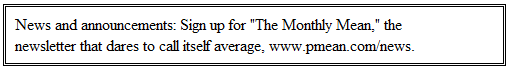P.Mean: Where did you get that formula for the confidence interval? (created 2008-09-09).I sent someone a confidence interval for a single proportion, and they asked how I computed it. That's a fair question. It turns out that I used a classic formula that everyone learns (and then forgets) in their basic Statistics class.

You can find webpages that explain and calculate this confidence interval for you, and I have a simple Excel spreadsheet that will also do the job. Go to the following page and jump down to the section labeled spreadsheets.

A nice explanation of this interval (and several competing intervals) is on the Wikipedia site (look for the section labeled "Normal approximation interval").

I actually helped write a bit of this page (I'm simon66217). The classic formula isNow some people do not like this formula, and for good reason. In certain situations, it can produce a lower limit less than zero or an upper limit greater than one. The recommendation, for example, of the Engineering Statistics Handbook The National Institute for Standards and Technology is to use a slightly more complex interval known as the Wilson interval.

This formulais a bit tedious, but it produces more accurate limits especially with small sample sizes combined with proportions very close to zero or one. There are other formulas, of course. Statistics is like real life. There a simple way to do things and an accurate way to do things, but the two rarely coincide.This work is licensed under a Creative Commons Attribution 3.0 United States License. This page was written by Steve Simon and was last modified on 2010-04-01. Need more information? I have a page with general help resources. You can also browse for pages similar to this one at Category: Confidence intervals.